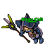## Wednesday, March 24, 2021

### TibiaGoals Riddles E7R2

Comment down below!

1.Move the arrow from the bottom right of the three to the left side to make it 2x4
Xarkost Eilia

2.Nehkz
Relania

Using one arrow of the 3 and make a 2 out of it.
2x4=8

3.Nehkz
Relania

Using one arrow of the 3 and make a 2 out of it.
2x4=8

4.Nehkz
Relania

Using one arrow of the 3 and make a 2 out of it.
2x4=8

5.2x4=8 (Dark Bombox)

6.1.By moving arrow on number 3
2x4=8
Eternal hunger
Antica

7.8.change 3 to 2 by moving down/right arrow to down/left

Impure

9.2*4=8 ashaer furmind

10.Só mudar a perna de baixo do número 3 de lado, fica 2 x 4 = 8. Nick: boxtop

11.Só mudar a perna de baixo do número 3 de lado, fica 2 x 4 = 8. Nick: boxtop

12.2 x 4 = 8

Shizurie

13.We will only move an arrow from the base of 3 and turn it into a number 2, the equation would be 2*4=8

2*4=8

14.2x4 =8
Aribebech

15.2 × 4 = 8
Mian Stone'arrow

16.17.Take the right down arrow that forms a 3 and move it to the left to form a 2.
So it's 2 x 4 = 8

Char: Marulina

18.Respuesta 2*4=8 ..
. Bebitha consentida

19.Moving the lower-right arrow of number three to the lower left now turns the equation into "2x4=8" :)

20.2x4=8
(moving 1 arrow from 3 to left-down side)

/Diego El Might

21.2 * 4 = 8 Dorrohav

22.23.Aurora Ventresca
2*4=8
Move second vertical arrow in number 3.

24.Só mudar a perninha de baixo do 3 pra fazer o 3 virar um 2. Fica 2 x 4 = 8. Nick: Boxtop

25.26.The equation true by moving only one arrow is 2x4=8
Char name: Fyori

27.Neo

Move the bottom vertical arrow to the left to make it 2x4 = 8

28.R= 2 x 4 = 8

Samuray de carro ;)

29.R= 2 x 4 = 8

Samuray de carro ;)

30.R= 2 x 4 = 8

Samuray de carro ;)

31.2 x 4 = 8
Moving bottom right vertical arrow on the '3' to the left to create a '2'

Boybarian
(firmera)

32.Zuali
https://i.gyazo.com/7a6e9e12f476cc1ce6acbe83407a8bb0.png

33.34.35.Character - Ragge Storm

To make the equation true, you need to move the arrow which is in the middle of the number 3. In particular it should be moved like this "I". Consequently, you wud have the number "2", so that, the equation could be possible.

36.2x4=8
Ray Jek

37.Turn the 3 into 2
2 x 4 = 8

Char: Dyaniixz

38.2x4=8
Character : Pyzaa / Antica

39.40.2x4=8 - Mikolaj

41.Answer: 2 x 4 = 8 /Norelli

42.Respuesta : 2 x 4 : 8 el 3 se convierte en 2 ...................... zazy torturador sombrio

43.2 x 4 = 8
Character: Amy Meow

44.Take one arrow from number 3 and move it to the left to make a 2
2 x 4 = 8

Skyelie Hasulhoff

45.You move the arrow on the bottom right of the 3 to the left making it 2 times 4 = 8
Toxic Hasulhoff

46.3 change to 2. the botom vertical arrow switch one position left. 2 x 4 = 8

1.My nick Procente

47.This comment has been removed by the author.

48.2x4=8
PAMELIIN

49.Myo Disturbed

the answer is by moving the lower right side arrow from the 3 to the left to make it a 2 x 4 = 8

50.change the 3 for 2 (2x4=8) Knight Narco

51.By moving arrow on number 3
2x4=8
Eternal hunger
Antica

52.By moving arrow on number 3
2x4=8
Eternal hunger
Antica

53.2x4=8
Just take bottom fight arrow from numer there to left side
Blood Evan

54.2x4=8
Just take bottom right arrow from numer there to left side
Blood Evan

55.2x4=8
Lovely Olka

56.By moving the arrow from the bottom right of the three to its left side to make it into a 2x4=8
My character's name is Bassyu
♥♥♥

57.By moving the arrow from the bottom right of the three to its left side to make it into a 2x4=8
My character's name is Bassyu
♥ ♥ ♥